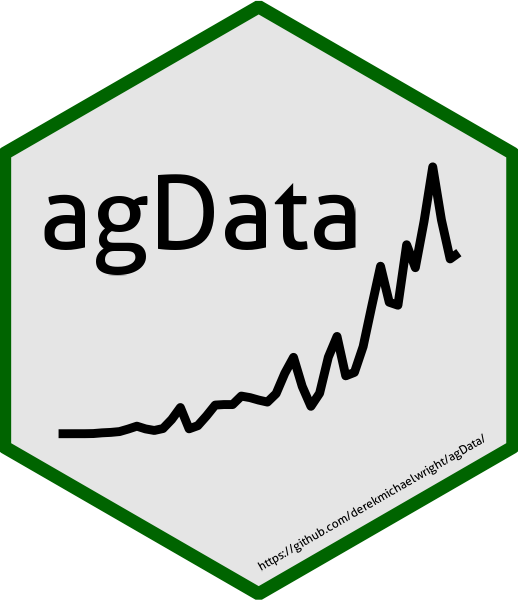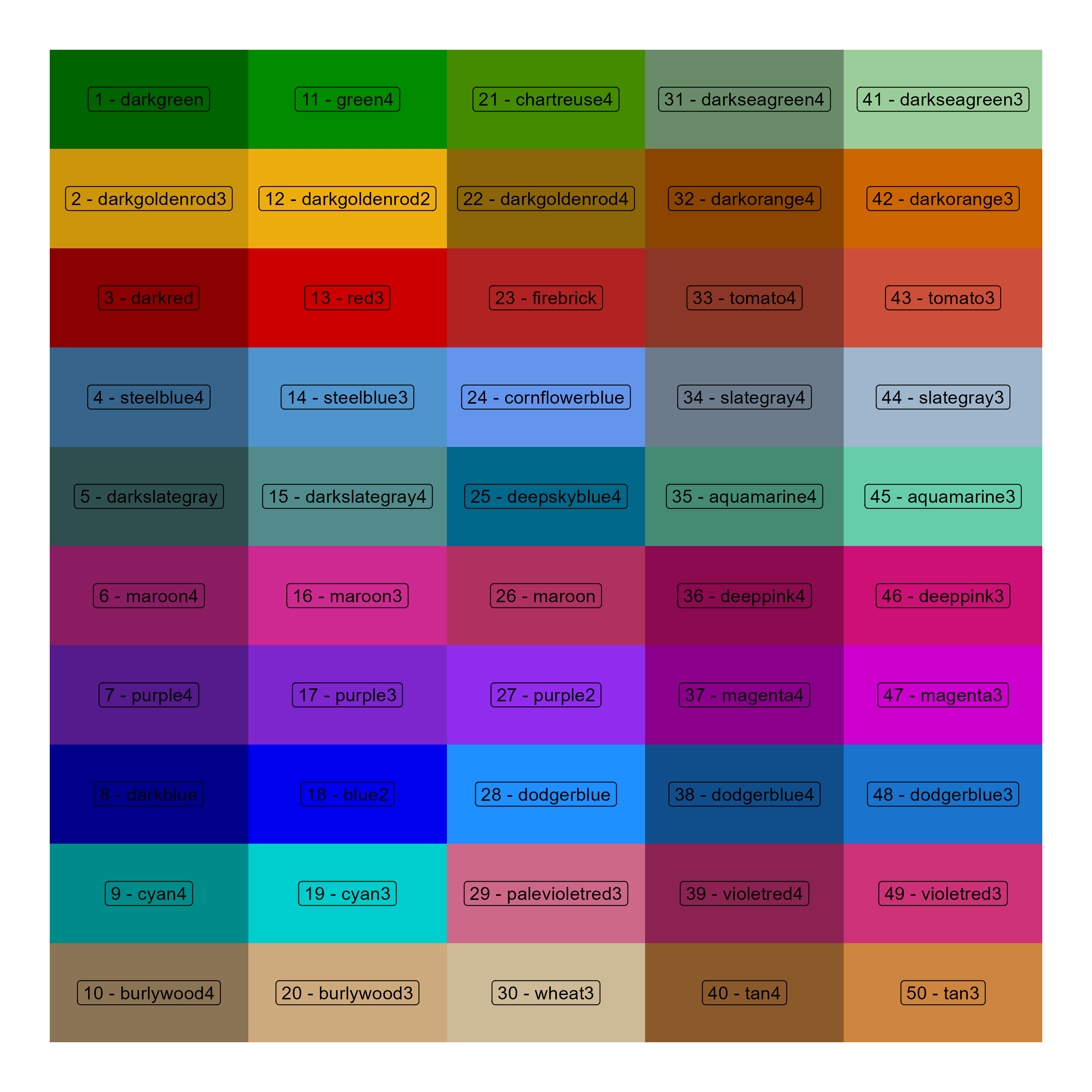agData: an R package containing various agricultural data sets

# Installation

devtools::install_github("derekmichaelwright/agData")
library(agData)# Data Sources

• FAO Food and Agriculture Organization of the United Nations fao.org/faostat/
• STATCAN Statistics Canada statcan.gc.ca/
• USDA United States Department of Agriculture usda.gov/
• ISAAA International Service for the Acquisition of Agri-biotech Applications isaaa.org/

# agData_Colors# Prep data
xx <- data.frame(
Color = factor(agData_Colors, levels = agData_Colors),
Number = 1:length(agData_Colors),
Row = rep(1:10, each = 10, length.out = length(agData_Colors)),
Col = rep(1:10, times = 10, length.out = length(agData_Colors)) )
# Plot
mp <- ggplot(xx, aes(x = Row, y = Col)) +
geom_tile(aes(fill = Color)) +
geom_label(aes(label = paste(Number, Color, sep = " - "))) +
scale_fill_manual(values = agData_Colors) +
scale_y_reverse() +
theme_void() +
theme(legend.position = "none")
ggsave("man/figures/agData_Colors.png", mp, width = 9, height = 9)